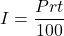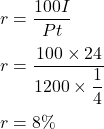## Use the formula for simple interest, I = Prt, to find the indicated quantity. Assume a 360 day year. 1 = $24; P =$1200; t = 90 days;

Question

Use the formula for simple interest, I = Prt, to find the indicated quantity. Assume a 360 day year.
1 = $24; P =$1200; t = 90 days; r = ?

in progress 0
1 month 2021-08-16T05:56:18+00:00 1 Answers 0 views 0

r = 8%

Step-by-step explanation:

Given that,

Interest, I = $24 Principal, P =$1200

Time, t = 90 days = 90/360 = 1/4 years

We need to find the rate.

We know that,

The simple interest is given by :Put all the values,So, the rate is 8%.